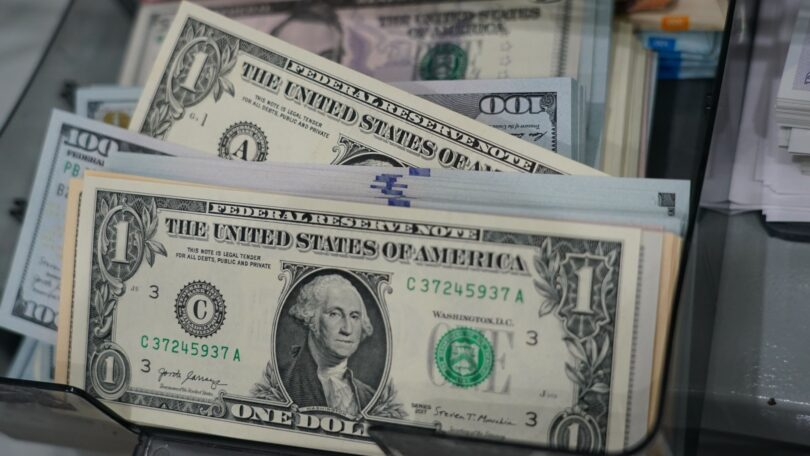# Money Economics Quiz## Commodity Money Economics quiz:

Ques. Which one of the following is related to commodity money?
(a) Stones
(b) Cattles
(c) Grains
(d) All of the above

Ans. (d)

Ques. Which of the following is not an element of high-powered money?
(a) Currency
(b) Repos
(c) Required Reserves
(d) Excess reserves

Ans. (b)

Ques. Consider the following statements: The price of any currency on the international market is decided by the

1. World Bank
2. demand for goods/services provided by the country concerned
3. stability of the government of the concerned country
4. economic potential of the country in question

Which of the statements given above are correct?
(a) 1, 2, 3 and 4
(b) 2 and 3 only
(c) 3 and 4 only
(d) 1 and 4 only

Ans. (b)

Ques. People are said to be suffering from “money illusions” since
(a) People merely consider nominal money holdings
(b) People consider real money balances
(c) Both A and B
(d) None of the above

Ans. (a)

Ques. In the case of ‘full-bodied money’
(a) Intrinsic value is less than face value
(b) Intrinsic value is equal to face value
(c) Intrinsic value is greater than face value
(d) None of the above

Ans. (b)

Related: Banking quiz

Ques. Which of the following is not related to commodity money?
(a) All commodities were not uniform in quality
(b) It is difficult to store and prevent the loss of value
(c) They lacked portability
(d) There was no problem with coincidence of wants

Ans. (c)

Ques. In the Quantity Theory of Money, Fischer states that, while other things remain the same,
(a) The price level varies directly with the quantity of money
(b) The price level varies inversely with the quantity of money
(c) Value of money varies directly with the quantity of money
(d) None of the above

Ans. (a)

Ques. Find the odd man out with reference to money
(a) Copper
(b) Silver
(c). Cattles
(d) Gold

Ans. (d)

Ques. Which one of the following is an example of “fiat money”?
(a) Precious stones
(b) Grains
(c) Gold coins
(d) Currency notes

Ans. (d)

Related: types of Cheque

Ques. Which of the following is the primary function of money?
(a) Measure of value
(c) Standard of deferred payments
(b) Store of value
(d) Basis of credit

Ans. (a)

Ques. The quantity theory of money was developed by:
(a) Marshall
(b) Ricardo
(b) Keynes
(d) Fisher

Ans. (d)

Ques. The ratio of money supply to high-powered money is:
(a) SLR
(b) CRR
(c) Money Multiplier
(d) Bank rate

Ans. (c)

Ques. Only Money Matters is Propounded by:
(a) Keynesians
(b) Monetarists
(c) Classicals
(d) Neo-Classicals

Ans. (b)

Ques. In the case of paper currency
(a) Intrinsic value and face value are equal
(b) Intrinsic value is less than face value
(c) Intrinsic value is greater than face value
(d) None of the above

Ans. (b)

Ques. Demand for money arises from
(a) Money acts as a medium of exchange
(b) Money acts as a store of value
(c) Both A and B
(d) Neither A nor B

Ans. (c)

Ques. __ is not an example of ‘near money’.
(a) Bill of exchange
(c) Bonds
(b) Treasury bills
(d) Currency notes

Ans. (d)

Ques. Which of the following measures would result in an increase in the money supply in the economy?

1. Purchase of government securities from the public by the Central Bank.
2. Deposit ‘of currency in commercial banks by the public
3. Borrowing by the government from’ the Central Bank
4. Sale of government securities to the public by the Central Bank

Select the correct answer using the codes given below:
(a) 1 only
(b) 2 and 4 only
(c) 1 and 3
(d) 2, 3 and 4

Ans. (c)